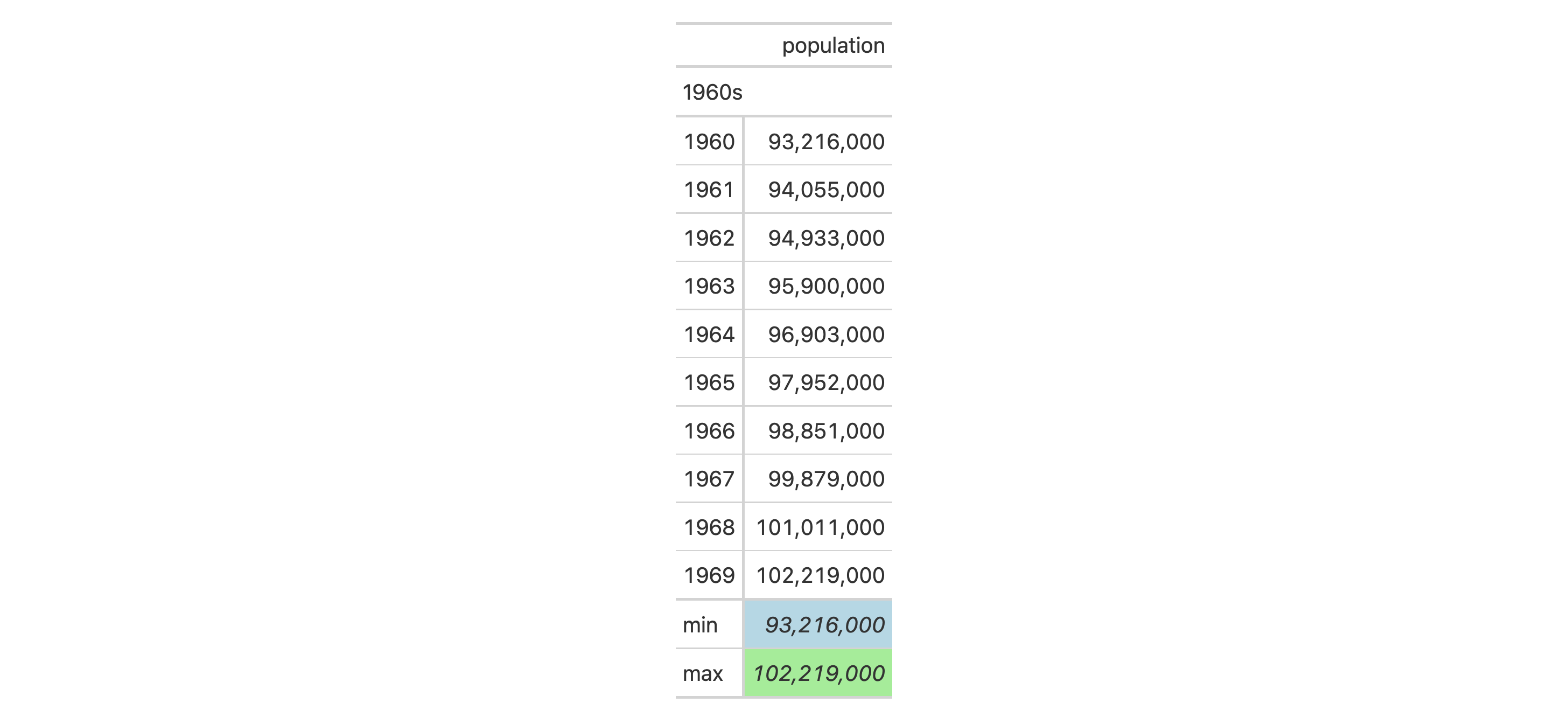# cells_summary: Location helper for targeting group summary cells In gt: Easily Create Presentation-Ready Display Tables

 cells_summary R Documentation

## Location helper for targeting group summary cells

### Description

The `cells_summary()` function is used to target the cells in a group summary and it is useful when applying a footnote with `tab_footnote()` or adding a custom style with `tab_style()`. The function is expressly used in each of those functions' `locations` argument. The 'summary' location is generated by the `summary_rows()` function.

### Usage

``````cells_summary(
groups = everything(),
columns = everything(),
rows = everything()
)
``````

### Arguments

 `groups` The names of the groups that the summary rows reside in. `columns` The names of the columns that are to be targeted. `rows` The names of the rows that are to be targeted.

### Value

A list object with the classes `cells_summary` and `location_cells`.

### Targeting cells with `columns`, `rows`, and `groups`

Targeting of summary cells is done through the `groups`, `columns`, and `rows` arguments. By default `groups` is set to `everything()`, which means that all available groups will be considered. Providing the ID values (in quotes) of row groups in `c()` will serve to constrain the targeting to that subset of groups.

The `columns` argument allows us to target a subset of summary cells contained in the resolved columns. We say resolved because aside from declaring column names in `c()` (with bare column names or names in quotes) we can use tidyselect-style expressions. This can be as basic as supplying a select helper like `starts_with()`, or, providing a more complex incantation like

`where(~ is.numeric(.x) && max(.x, na.rm = TRUE) > 1E6)`

which targets numeric columns that have a maximum value greater than 1,000,000 (excluding any `NA`s from consideration).

Once the groups and columns are targeted, we may also target the `rows` of the summary. Summary cells in the stub will have ID values that can be used much like column names in the `columns`-targeting scenario. We can use simpler tidyselect-style expressions (the select helpers should work well here) and we can use quoted row identifiers in `c()`. It's also possible to use row indices (e.g., `c(3, 5, 6)`) that correspond to the row number of a summary row in a row group (numbering restarts with every row group).

### Overview of location helper functions

Location helper functions can be used to target cells with virtually any function that has a `locations` argument. Here is a listing of all of the location helper functions, with locations corresponding roughly from top to bottom of a table:

• `cells_title()`: targets the table title or the table subtitle depending on the value given to the `groups` argument (`"title"` or `"subtitle"`).

• `cells_stubhead()`: targets the stubhead location, a cell of which is only available when there is a stub; a label in that location can be created by using the `tab_stubhead()` function.

• `cells_column_spanners()`: targets the spanner column labels with the `spanners` argument; spanner column labels appear above the column labels.

• `cells_column_labels()`: targets the column labels with its `columns` argument.

• `cells_row_groups()`: targets the row group labels in any available row groups using the `groups` argument.

• `cells_stub()`: targets row labels in the table stub using the `rows` argument.

• `cells_body()`: targets data cells in the table body using intersections of `columns` and `rows`.

• `cells_summary()`: targets summary cells in the table body using the `groups` argument and intersections of `columns` and `rows`.

• `cells_grand_summary()`: targets cells of the table's grand summary using intersections of `columns` and `rows`

• `cells_stub_summary()`: targets summary row labels in the table stub using the `groups` and `rows` arguments.

• `cells_stub_grand_summary()`: targets grand summary row labels in the table stub using the `rows` argument.

• `cells_footnotes()`: targets all footnotes in the table footer (cannot be used with `tab_footnote()`).

• `cells_source_notes()`: targets all source notes in the table footer (cannot be used with `tab_footnote()`).

When using any of the location helper functions with an appropriate function that has a `locations` argument (e.g., `tab_style()`), multiple locations can be targeted by enclosing several `⁠cells_*()⁠` helper functions in a `list()` (e.g., `list(cells_body(), cells_grand_summary())`).

### Examples

Use `countrypops` to create a gt table. Add some styling to the summary data cells with with `tab_style()`, using `cells_summary()` in `locations`.

```countrypops |>
dplyr::filter(country_name == "Japan", year < 1970) |>
dplyr::select(-contains("country")) |>
dplyr::mutate(decade = paste0(substr(year, 1, 3), "0s")) |>
gt(
rowname_col = "year",
) |>
fmt_number(
columns = population,
decimals = 0
) |>
summary_rows(
groups = "1960s",
columns = population,
fns = list("min", "max"),
fmt = ~ fmt_integer(.)
) |>
tab_style(
style = list(
cell_text(style = "italic"),
cell_fill(color = "lightblue")
),
locations = cells_summary(
groups = "1960s",
columns = population,
rows = 1
)
) |>
tab_style(
style = list(
cell_text(style = "italic"),
cell_fill(color = "lightgreen")
),
locations = cells_summary(
groups = "1960s",
columns = population,
rows = 2
)
)
```8-13

### Function Introduced

`v0.2.0.5` (March 31, 2020)

Other helper functions: `adjust_luminance()`, `cell_borders()`, `cell_fill()`, `cell_text()`, `cells_body()`, `cells_column_labels()`, `cells_column_spanners()`, `cells_footnotes()`, `cells_grand_summary()`, `cells_row_groups()`, `cells_source_notes()`, `cells_stub_grand_summary()`, `cells_stub_summary()`, `cells_stubhead()`, `cells_stub()`, `cells_title()`, `currency()`, `default_fonts()`, `escape_latex()`, `google_font()`, `gt_latex_dependencies()`, `html()`, `md()`, `pct()`, `px()`, `random_id()`, `stub()`, `system_fonts()`